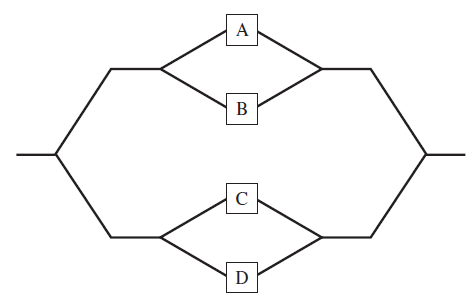×
Get Full Access to Statistics For Engineers And Scientists - 4 Edition - Chapter 2 - Problem 1se
Get Full Access to Statistics For Engineers And Scientists - 4 Edition - Chapter 2 - Problem 1se

×

# A system consists of four components connected as shown.ISBN: 9780073401331 38

## Solution for problem 1SE Chapter 2

Statistics for Engineers and Scientists | 4th Edition

• Textbook Solutions
• 2901 Step-by-step solutions solved by professors and subject experts
• Get 24/7 help from StudySoup virtual teaching assistantsStatistics for Engineers and Scientists | 4th Edition

4 5 1 264 Reviews
16
0
Problem 1SE

A system consists of four components connected as shown.Assume A, B, C, and D function independently. If the probabilities that A, B, C, and D fail are 0.1, 0.2, 0.05, and 0.3, respectively, what is the probability that the system functions?

Step-by-Step Solution:

Solution :

Given, a system consists four component A,B,C and D.

From the given diagram

A be the event that component function is A.

B be the event that component function is B.

C be the event that component function is C and

D be the event that component function is D.

Here we assumed independently function is A,B,C and D.

Our goal is to find

a). What is the probability that the system functions?

Step 1 of 1 :

If the probability fails

A=0.1, B=0.2, C=0.05 and C=0.3.

Then,

P(A) = 1-0.1

P(A) = 0.9

P(B) = 1-0.2

P(B) = 0.8

P(C) = 1-0.05

P(C) = 0.95 and

P(D) = 1-0.3

P(D) = 0.7

Now we have to find the probability that the system functions.

From the diagram 3 subsystem is there.ThereforeWe have to substitute the value.ThereforeWe have to substitute the value.Therefore.

Conclusion :andStep 2 of 1

##### ISBN: 9780073401331

Since the solution to 1SE from 2 chapter was answered, more than 640 students have viewed the full step-by-step answer. The answer to “?A system consists of four components connected as shown. Assume A, B, C, and D function independently. If the probabilities that A, B, C, and D fail are 0.1, 0.2, 0.05, and 0.3, respectively, what is the probability that the system functions?” is broken down into a number of easy to follow steps, and 42 words. Statistics for Engineers and Scientists was written by and is associated to the ISBN: 9780073401331. This full solution covers the following key subjects: system, functions, connected, consists, fail. This expansive textbook survival guide covers 153 chapters, and 2440 solutions. The full step-by-step solution to problem: 1SE from chapter: 2 was answered by , our top Statistics solution expert on 06/28/17, 11:15AM. This textbook survival guide was created for the textbook: Statistics for Engineers and Scientists , edition: 4.

Unlock Textbook Solution